Concepts of Biology (BIOL115) - Dr. S.G. Saupe (ssaupe@csbsju.edu); Biology Department, College of St. Benedict/St. John's University, Collegeville, MN 56321

Spuds McSaupe Plays with Peroxide

Today our friendly tuber-tester, Spuds McSaupe, will demonstrate enzyme action.  The reaction we will study concerns the breakdown of hydrogen peroxide into water and oxygen.  We can diagram this reaction as follows:

2H2O2  → O2 + 2H2O

In vivo (which means in the living cell rather than in a test tube = in vitro), this reaction is catalyzed by the enzyme catalase (technical name: hydrogen peroxide oxidoreductase).

Q1.  What is the substrate for this enzyme? ________________________________
Q2.  What is (are) the product(s) of this reaction? ___________________________
Q3   What kind of reaction does this enzyme catalyze? _______________________

The cells of many organisms contain catalase.

Q3.  In what organelle(s) does catalase typically occur? ______________________

But, what about potatoes?  Do potato cells contain catalase?  What do you think?

Hypothesis:  I think that catalase (does / doesn't) occur in potato cells because (write your rationale for hypothesis):

Predictions:  (complete the following)

• IF potato cells contain catalase, THEN when I put potato cores in water they (will / will not) foam.

• IF potato cells contain catalase, THEN when I put potato cores in peroxide they (will / will not) foam.

• IF potato cells contain catalase, THEN when I put heated potato cores in peroxide they (will / will not) foam.

Experimental Protocol:

1. Obtain two potatoes.  Punch holes in the skin of one and then microwave for 20 seconds or longer (depending on size)

2. With a cork borer, prepare cores from both tubers

3. Place one potato core in each of the treatments listed in Table 1.

4. Record whether foaming/bubbling occurs (-, +, +++)

 Table 1.  Effect of treating potato cores with various solutions Treatment Results (foaming: -, +, +++) 1. Water (no potato) 2. Water + potato core (fresh) 3. Hydrogen peroxide (no potato) 4. Hydrogen peroxide + potato core (fresh) 5. Hydrogen peroxide + microwaved potato core

Results/Conclusions:

1. Do these results support/refute your hypothesis about the occurrence of catalase in potato cells?

2. How do we know that in our experiment foaming is the result of catalase activity and not a non-enzymatic breakdown of hydrogen peroxide?

3. Why do the solutions foam?  Describe a way that you could test this hypothesis.

4. Hydrogen peroxide slowly breaks down over time and looses its potency.  What does this suggest about the ΔG of the reaction?

5. The breakdown of hydrogen peroxide is a spontaneous reaction:  a.  False    b.  True

6. The breakdown of hydrogen peroxide is an endergonic reaction:  a.  False   b.  True

7. Why do potato cells and other types of cells produce catalase?  In other words, what is the function of catalase?

8. Suppose we want to measure the rate (amount of oxygen in mMol produced per minute) of catalase activity.  To do so, we could catch any oxygen released during foaming and then measure the amount of oxygen produced over time.  We would then plot a graph of the amount of product formed (mMol) vs. time (min).  To determine the rate we would calculate the slope of the line. Calculate the rate of the reaction below.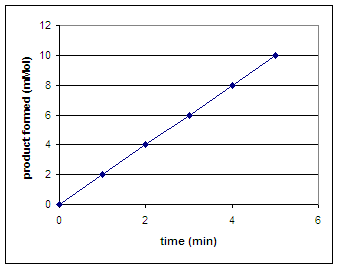9. Suppose we wanted to investigate the impact of enzyme concentration on the rate of the reaction.  We would repeat our experiment using several different concentrations of enzyme but not vary the substrate concentration.  On the graph on the left below, sketch the lines that you would expect to result if you repeated the experiment using 1, 2, 3, 4, and 6 units of the enzyme.  To calculate the actual rate of the reaction at each enzyme concentration, we can determine the slope of each of the lines on our graph.  Then, on the graph on the right sketch in the line that would result if you plot the rate of each reaction vs. enzyme concentration (0 - 6 units).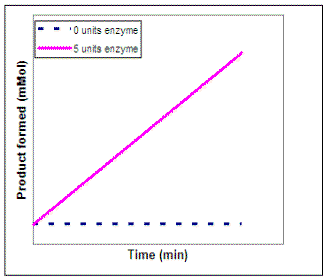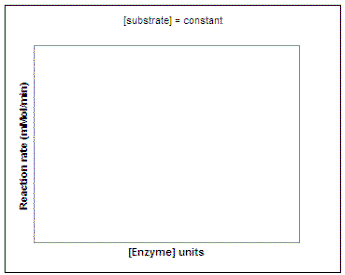10. Now suppose we wanted to investigate the impact of substrate concentration on the rate of the reaction.  We would repeat our experiment using several different concentrations of substrate but not vary the enzyme concentration.  On the graph on the left below, sketch the lines that you would expect to result if you repeated the experiment using 1, 2, 3, 4, 6, and 10 units of the substrate.  Then, on the graph on the right sketch in the line that would result if you plot the rate of each reaction vs. substrate concentration (0 - 10 units).  Draw a line on the graph below to show the predicted results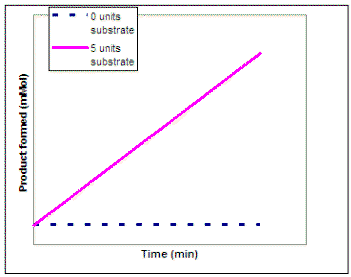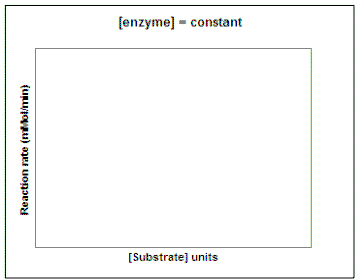1. Let's repeat this experiment using a constant amount of enzyme and substrate and determine the reaction rate at different temperatures and pH values.  In the graphs below, sketch in the predicted result.  (Question to ponder - what do you predict the pH and temperature optimum for this enzyme will be in potatoes?)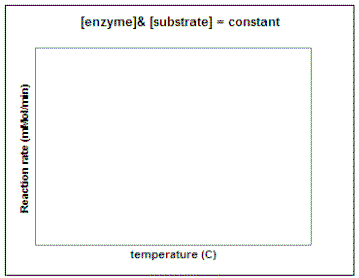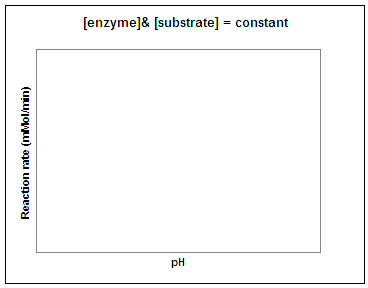2. Why do you put hydrogen peroxide on wounds?

 | Top | SGS Home | CSB/SJU Home | Biology Dept | Biol115  Section Home Page | Concepts Home Page | Disclaimer |

Last updated: September 19, 2006     � Copyright by SG Saupe / URL:http://www.employees.csbsju.edu/ssaupe/index.html